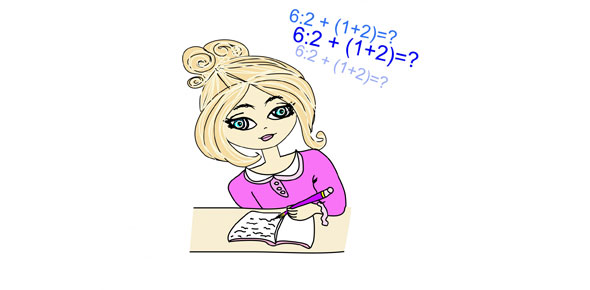# Maths SAT Preparations Trivia Questions

11 Questions | Attempts: 102
ShareSettingsTaking your SATs soon, huh? That can be a pretty stressful time in a person’s life, but we’re here to help you ensure that you’re good to go when the time comes. Let’s take a look at your math skills!

• 1.
If four apples cost 20 cents, then, at this rate, how much would ten apples cost?
• A.

\$.40

• B.

\$.50

• C.

\$.60

• D.

\$.70

• E.

\$.80

• 2.
The first day of a particular month is Tuesday. What day of the week will it be on the 31st day of the month?
• A.

Wednesday

• B.

Thursday

• C.

Friday

• D.

Saturday

• E.

Sunday

• 3.
How many integer pairs (m,n) satisfy the statements 0< m + n < 50, and m/n equals 8?
• A.

5

• B.

6

• C.

7

• D.

8

• E.

More than 8

• 4.
If y% of 50 is 32, then what is 200% of y?
• A.

16

• B.

32

• C.

64

• D.

128

• E.

256

• 5.
In the following sequence:-1,1,2,-1,1,2,-1,1,2What is the sum of the first 25 terms?
• A.

15

• B.

16

• C.

18

• D.

19

• E.

21

• 6.
A jar contains only white and blue marbles of identical size and weight.  The ratio of the number of white marbles to the number of blue marbles is 4 to b. If the probability of choosing a white marble from the jar at random is 1/4, then what is the value of b?
• A.

1

• B.

2

• C.

6

• D.

12

• E.

16

• 7.
The area of a right triangle is 10 square centimeters. If the length of each leg, in centimeters, is a positive integer, then what is the least possible length, in centimeters, of the hypotenuse?
• A.

Square root of 29

• B.

Square root of 41

• C.

Square root of 101

• D.

Square root of 104

• E.

Square root of 401

• 8.
If y is a number less than 0 but greater than -1, which of the following expressions has the greatest value?
• A.

100y

• B.

Y^2

• C.

Y^3

• D.

Y^4

• E.

Y^5

• 9.
If at least one wuzzle is grumpy, then some fuzzles are lumpy.If the statement above is true, then which of the following must also be true?
• A.

If all wuzzles are grumpy, then all fuzzles are lumpy.

• B.

If no wuzzle is grumpy, then all fuzzles are lumpy.

• C.

If all fuzzles are lumpy, then all wuzzles are grumpy.

• D.

If no wuzzle is grumpy, then no fuzzle is lumpy.

• E.

If no fuzzle is lumpy, then no wuzzle is grumpy.

• 10.
The volume of a right cylinder A is twice the volume of a right cylinder B. If the height of cylinder B is twice the height cylinder A, then what is the ratio of the radius of cylinder A to the radius of cylinder B?
• A.

1 to 2

• B.

1 to 1

• C.

Square root of 2, to 1

• D.

2 to 1

• E.

4 to 1

• 11.
In a set of 30 different positive integers, every number is even and/or a multiple of 3. If 22 of these numbers are even and 15 are multiples of 3, then how many of these numbers are multiples of 6?
• A.

23

• B.

22

• C.

15

• D.

11

• E.

7

## Related TopicsBack to top
×

Wait!
Here's an interesting quiz for you.